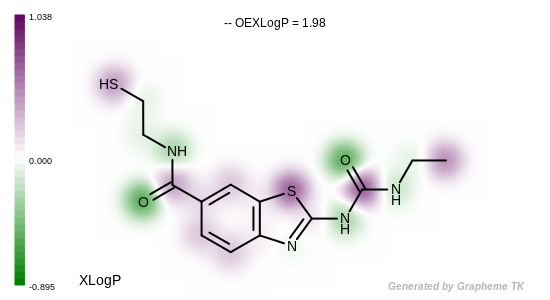OEGetXLogP¶

bool OEGetXLogP(const OEChem::OEMolBase &mol, float &xlogp, float *atomxlogps=0)

Returns the XLogP for a given molecule as described in the LogP section. The returned value will be equal to the sum on the individual atom contributions plus the linear regression constant -0.127.

The atomxlogps parameter can be used to retrieve the contribution of each atom to the total XLogP as shown in Listing 2. See example in Figure: Example of depicting the atom contributions of the XLogP.

Listing 2: Example of retrieving individual atom contributions to XLogP

// declare an array for atom values
float * atomXLogP = new float[mol.GetMaxAtomIdx()];
float xlogp;
bool rc = OEGetXLogP(mol, xlogp, atomXLogP);

if (!rc)
std::cout << "XLogP failed for molecule" << std::endl;
else
{
std::cout << "XLogP = " << xlogp << std::endl;
for (OEIter<OEAtomBase> atom=mol.GetAtoms();atom;++atom)
{
unsigned int idx = atom->GetIdx();
std::cout << idx << " " << atomXLogP[idx] << std::endl;
}
}

// clean up
delete [] atomXLogP;Example of depicting the atom contributions of the XLogP

Hint

XLogP should be calculated on the neutral form of the molecule. Therefore, calling the OERemoveFormalCharge function of Quacpac TK is highly recommended prior to the XLogP calculation.